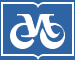## Издательство СО РАН

Адрес Издательства СО РАН: Россия, 630090, а/я 187
Новосибирск, Морской пр., 2# Главная – Журналы – Журнал структурной химии 2015 номер 5

## Журнал структурной химии

### 2015 год, номер 5

#### QSAR STUDIES OF HEPT DERIVATIVES AS ANTI-HIV DRUGS USING THE RASMS METHOD

J. Tong, X. Zhao, L. Zhong, J. Chang
Shaanxi University of Science and Technology, Xi'an, P. R. China
Ключевые слова: random sampling analysis on molecular surface (RASMS), HEPT derivatives, quantitative structure activity relationship (QSAR), multiple linear regression (MLR)
Страницы: 914-921
Подраздел: ТЕОРИЯ СТРОЕНИЯ МОЛЕКУЛ И ХИМИЧЕСКОЙ СВЯЗИ

Аннотация

Random sampling analysis on the molecular surface (RASMS) is used to describe the chemical structures of 35 HEPT derivatives as anti-HIV drugs. Here a quantitative structure activity relationship (QSAR) model is built by multiple linear regression (MLR). The estimation stability and prediction ability of the model are strictly analyzed by both internal and external validations. The correlation coefficients of the established MLR model, leave-one-out (LOO) cross-validation, and predicted values versus experimental ones of external samples were r 2 = 0.851, = 0.746, and r 2(test) = 0.815 respectively. The satisfactory results show that RASMS can express the information related to the biological activity of HEPT derivatives.

DOI: 10.15372/JSC20150506